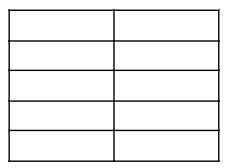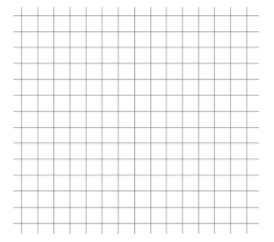# Equations and Inequalities

## Objective

Represent the relationship between two quantities in graphs, equations, and tables. (Part 2)

## Common Core Standards

### Core Standards

?

• 6.EE.C.9 — Use variables to represent two quantities in a real-world problem that change in relationship to one another; write an equation to express one quantity, thought of as the dependent variable, in terms of the other quantity, thought of as the independent variable. Analyze the relationship between the dependent and independent variables using graphs and tables, and relate these to the equation. For example, in a problem involving motion at constant speed, list and graph ordered pairs of distances and times, and write the equation d = 65t to represent the relationship between distance and time.

• 6.RP.A.3.A — Make tables of equivalent ratios relating quantities with whole number measurements, find missing values in the tables, and plot the pairs of values on the coordinate plane. Use tables to compare ratios.

?

• 5.OA.B.3

• 6.NS.C.6.C

## Criteria for Success

?

1. Identify the independent and dependent variables in a relationship between two quantities.
2. Create a table of values for a relationship.
3. Plot values from a table on a coordinate plane.
4. Write and identify equations to represent the relationship between two associated quantities.

## Tips for Teachers

?

Lessons 13 and 14, similar to Lesson 12, bring together several concepts from standards such as 6.RP.3, 6.EE.7, and 6.NS.8 to represent relationships between quantities in different ways. In this lesson, students look at nonproportional relationships in the form $x+p=q$.

#### Fishtank Plus

• Problem Set
• Student Handout Editor
• Vocabulary Package

## Anchor Problems

?

### Problem 1

Jordan is 2 years older than her sister Nora. Let $j$ represent Jordan’s age and $n$ represent Nora’s age.

1. Write an equation to represent the relationship between the ages of the two sisters.
2. Create a table of values using your equation.
3. Plot the points in the coordinate plane.### Problem 2

The table below shows the output values for different input values.

 Input ($x$) Output ($y$) 1 6 2 11 3 16 4 21

Which equation represents the relationship between the inputs and outputs?

1.   ${x+5=y}$
2.   ${x+9=y}$
3.   ${4x+3=y}$
4.   ${5x+1=y}$

### Problem 3

Zoe is collecting books to donate. She started with 3 books and collects 2 more each week. She is using the equation ${b=2w+3}$, where $b$ is the total number of books collected and $w$ is the number of weeks she has been collecting books.

1. Name the independent and dependent variables.
2. Create a table of values and then use it to graph Zoe’s book collection.#### References

EngageNY Mathematics Grade 6 Mathematics > Module 4 > Topic H > Lesson 32Exercise 2

Grade 6 Mathematics > Module 4 > Topic H > Lesson 32 of the New York State Common Core Mathematics Curriculum from EngageNY and Great Minds. © 2015 Great Minds. Licensed by EngageNY of the New York State Education Department under the CC BY-NC-SA 3.0 US license. Accessed Dec. 2, 2016, 5:15 p.m..

Modified by The Match Foundation, Inc.

## Problem Set

?The following resources include problems and activities aligned to the objective of the lesson that can be used to create your own problem set.

• Include review from other lessons in the unit, including fluency practice with solving equations and inequalities with decimals and fractions.

?

Mara and Evan work at the same company. Mara started working at the company 5 years before Evan started. Let $m$ represent the number of years Mara has worked at the company and $e$ represent the number of years Evan has worked at the company.

1. Write an equation to represent the relationship.
2. Create a table of values using your equation.
3. Plot the points in the coordinate plane.?